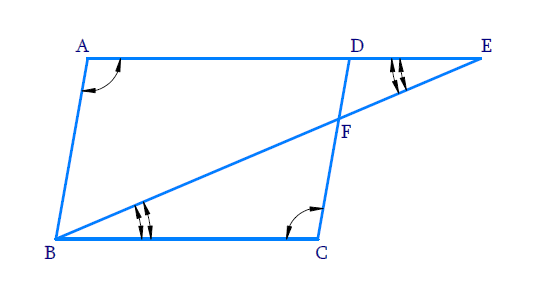# Ex.6.3 Q8 Triangles Solution - NCERT Maths Class 10

Go back to  'Ex.6.3'

## Question

$$E$$ is a point on the side $$AD$$ produced of a parallelogram $$ABCD$$ and $$BE$$ intersects $$CD$$ at $$F.$$ Show that $$\Delta ABE \sim \Delta CFB.$$

DiagramVideo Solution
Triangles
Ex 6.3 | Question 8

## Text Solution

Reasoning:

If two angles of one triangle are respectively equal to two angles of another triangle, then the two triangles are similar.

This may be referred to as the $$AA$$ similarity criterion for two triangles.

Steps:

In $$\Delta \rm{A B E}, \Delta \rm{C F B}$$

$$\angle B A E=\angle F C B$$

$$\text{(opposite angles of a parallelogram)}$$

$$\angle A E B=\angle F B C$$

\begin{align} \begin{bmatrix} \because AE \,||\, BC \; \text{ and } \; EB \\ \text{ is a transversal alternate angle}\end{bmatrix} \end{align}

$$\Rightarrow\;\, \Delta ABE \sim \Delta C F E$$

$$\text{(AA Criterion)}$$

Learn from the best math teachers and top your exams

• Live one on one classroom and doubt clearing
• Practice worksheets in and after class for conceptual clarity
• Personalized curriculum to keep up with school